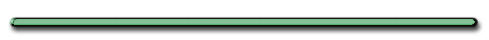The football has 2 velocities.
Which velocity is the football player matching.

Horizontal velocity.
The football and the football player
have a constant horizontal velocity.

 Ex) A projectile leaves ground at an angle of 60.° and a speed of 100.m/s. (a) Find the objects maximum height & total time in the air

(level ground)

Ex) A projectile leaves ground at an angle of 60.° and a speed of  100. m/s.

First find the initial vertical component of the object's velocity

Ө = 60.°

Vi  = 100. m/s
Viy = ?Viy = Vi(sinӨ)

= (100. m/s)sin60.°

Viy = 87. m/s up

(b) Find the objects maximum height.

Viy = 87. m/s
ay = -10. m/s2 (ascending)
dy (max) =?
Vfy = 0 (at max height)

02 = (87. m/s)2 + 2(-10. m/s2)d

0 = 7569 + -20.dy

subtract 7569 from both sides

-7569 = -20.(dy)

dy = 378 m

Actually ......

dy = 380 m

(2 sig. figs)

(b) Total time in the air

Viy = 87. m/s up

t = 2(87 m/s)/9.8 m/s2

t = 18 sec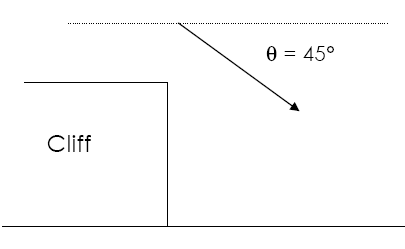ex) A rock is thrown from a cliff with initial speed of 40. m/s at an angle of 45.° below  the horizontal.  (a) If rock strikes ground in 1 sec, height of cliff?
`        `

a) What is the vertical component  of the initial velocity?Ө = 45.°

` `
`Vi = 40. m/s`
`Viy = ?     `
` `
`Viy = VsinӨ`
` `
`= (40. m/s)sin45.° `

` `
`Viy = 28 m/s down `

(b) If the rock strikes ground in 1.0 sec what is height of cliff?

dy = ? (height)

ay = + 10. m/s2

t = 1.0 sec

Viy = 28 m/s (from before)

`dy = Viyt + (½)at2`
` `
` `
` `
` `
` `
`= 28.m/s(1sec)+½(10. m/s2)(1.0sec)2`
` `
` `
` `
`28 m + 5.0 m`
` `

` `
`dy = 33 m down`
` `
` `
``
` `
` `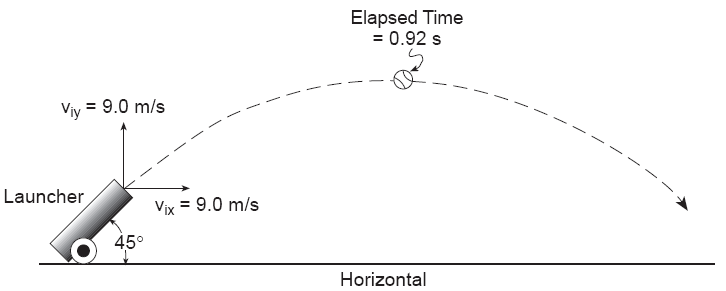At Peak,

Vx = ?Vx = 9.0 m/sh

Total Time in Air?

1.84 sec
(twice peak)

actually 1.8

(2 sig. figs.)What will the horizontal velocity of the baseball be just before it hits the ground?

9 m/s, Vx is constant

Ex) A wild bowler releases the his bowling ball at a speed of 15 m/s and an angle of 34.0 degrees above the horizontal.

Calculate the horizontal distance that the bowling ball travels if it leaves the bowlers hand at a height of 2.20 m above the ground.

dx = Vxt = Vcosqt

Need to find t

`dy = Viyt + (½)at2`

`Viy = Vsinq`
`Viy = 8.6 m/s`
` `
`dy = Viyt + (½)at2`
` `
`2.20 m = (-8.6m/s)t + 4.9t2`
`0 = 4.9t2 +(-8.6m/s)t - 2.20m`
` `
`Quadratic Equation`

`t = 1.99 s, -0.225 s`

dx = Vxt = Vcosqt

dx = Vxt

= (15m/s)cos34(1.99 sec)

=  25.6 m

The ammunition fired from an M-16 rifle has a muzzle velocity of 1000 m/s. A sniper perched on a tower 50 m high aims at a target and fires.

(A) The sniper aims her rifle 53.1 degrees above the horizontal. How far away from the platform does the bullet hit the ground?

Neglect air resistance.

Vx = VcosӨ = 600 m/s

dx = VcosӨt = [600m/s]t =

Need to find t

Make down positive

Δdy = -VsinӨt + 1/2gt2

50 m = -1000m/s[sin53.1]t + 4.9t2

50 m = -800t + 4.9t2

0 = 4.9t2 - 800t - 50. m

t = 163 sec

Vx = VcosӨ = 600 m/s

dx = VcosӨt = [600m/s]t =

dx = 98000 m

(B) With what speed would the bullet hit?

Vx = 600 m/s

down Neg.

Vfy = -VsinӨ + gt

Vfy = -800m/s + 10m/s2(163sec)

Vfy = 830 m/s

Pythagorean Theorem

V = 1000 m/s

(C) If the target is 500 meters away on a platform 50 meters high, what angle would the sniper have to make with her gun to hit the target?

Dx = Vxt

500 m = [(1000m/s)cosӨ]t

Δdy = Viyt + (½)at2

0 = Viyt + (½)at2

0 = VsinӨt + 5t2

(A) 98000 m (B) 1000 m/s (C) 44.9Monkey and Hunter
Don Ion

(offsite)Don Ion| Onsite |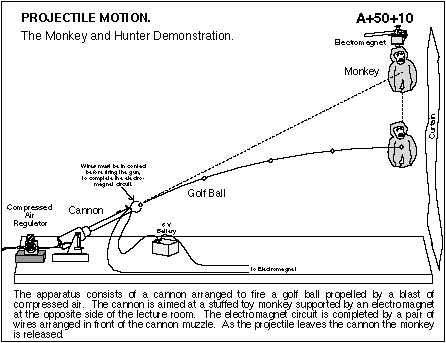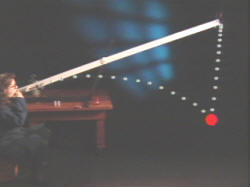Projectile Motion Game
St. Mary's Physics